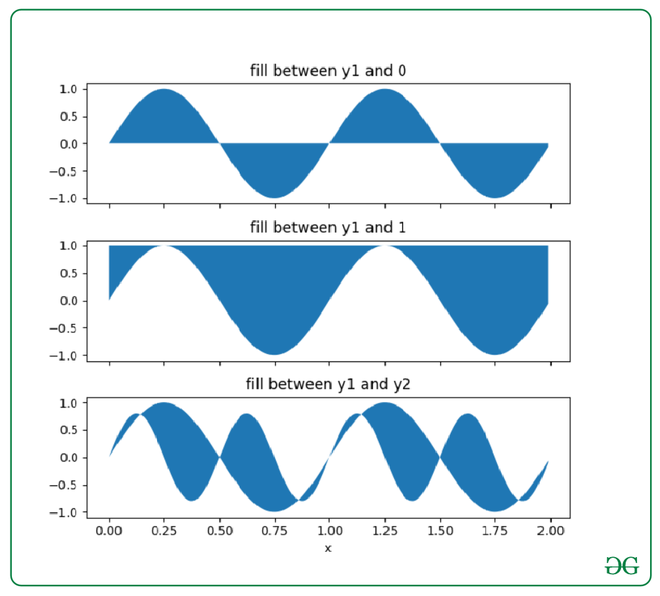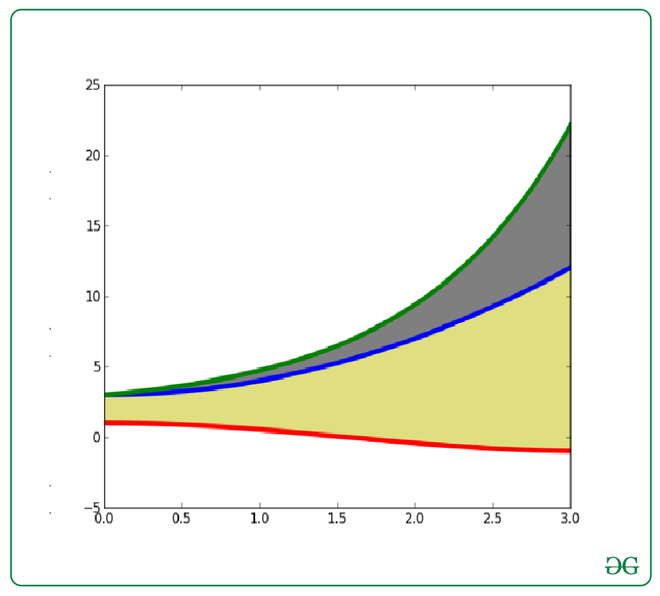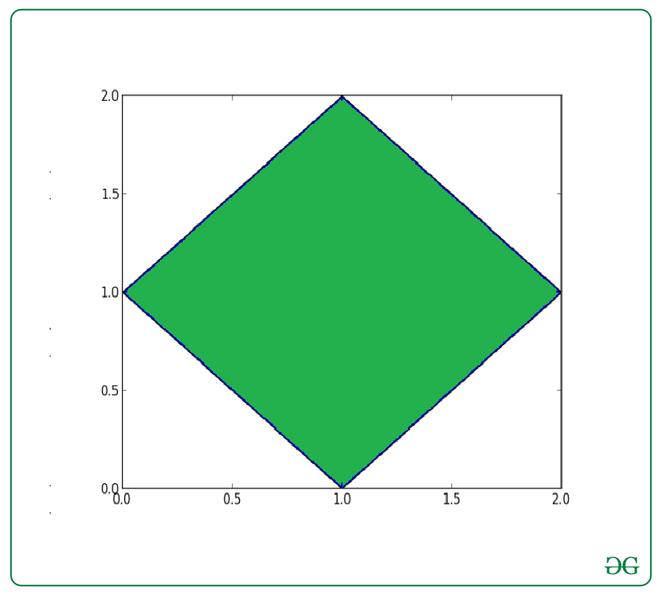# How to Fill Between Multiple Lines in Matplotlib?

With the use of the fill_between()  function in the Matplotlib library in Python, we can easily fill the color between any multiple lines or any two horizontal curves on a 2D plane.

Syntax: matplotlib.pyplot.fill_between(x, y1, y2=0, where=None, step=None, interpolate=False, *, data=None, **kwargs)

Example 1: Color between the curve of the mathematical function f(x)=sin(x)

## Python3

 `import` `pylab as plt` `import` `numpy as np`   `x ``=` `np.arange(``0.0``, ``2``, ``0.01``)` `y1 ``=` `np.sin(``2` `*` `np.pi ``*` `x)` `y2 ``=` `0.8` `*` `np.sin(``4` `*` `np.pi ``*` `x)`   `fig, (ax1, ax2, ax3) ``=` `plt.subplots(` `  ``3``, ``1``, sharex``=``True``, figsize``=``(``6``, ``6``))`   `ax1.fill_between(x, y1)` `ax1.set_title(``'fill between y1 and 0'``)`   `ax2.fill_between(x, y1, ``1``)` `ax2.set_title(``'fill between y1 and 1'``)`   `ax3.fill_between(x, y1, y2)` `ax3.set_title(``'fill between y1 and y2'``)` `ax3.set_xlabel(``'x'``)` `fig.tight_layout()`

Output:Example 2: Color between the curve of the mathematical function f(x)=cos(x) and f(x)=exp(x) :-

## Python3

 `import` `pylab as plt` `import` `numpy as np`   `X ``=` `np.linspace(``0``, ``3``, ``200``)` `Y1 ``=` `X``*``*``2` `+` `3` `Y2 ``=` `np.sin(X)` `Y3 ``=` `np.cos(X)`   `plt.plot(X, Y1, lw``=``4``)` `plt.plot(X, Y2, lw``=``4``)` `plt.plot(X, Y3, lw``=``4``)`   `plt.fill_between(X, Y1, Y2, color``=``'k'``, alpha``=``.``5``)` `plt.fill_between(X, Y1, Y3, color``=``'y'``, alpha``=``.``5``)`   `plt.show()`

Output:Example 3: Color the Rhombus :-

## Python3

 `import` `matplotlib.pyplot as plt`     `x ``=` `[``1``, ``2``, ``1``, ``0``]` `y ``=` `[``2``, ``1``, ``0``, ``1``]`   `plt.fill(x, y)` `plt.show()`

Output:Whether you're preparing for your first job interview or aiming to upskill in this ever-evolving tech landscape, GeeksforGeeks Courses are your key to success. We provide top-quality content at affordable prices, all geared towards accelerating your growth in a time-bound manner. Join the millions we've already empowered, and we're here to do the same for you. Don't miss out - check it out now!

Previous
Next